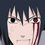# Pi Day

Share different ways to derive value of pie. For example one way is that Archimedes and others up to the end of the Middle Ages used to compute Pi was to approximate it using a regular polygon of n sides and its inscribed and circumscribed circles. The inscribed circle has circumference smaller than the perimeter of the polygon, which is in turn smaller than the circumference of the circumscribed circle. That gave inequalities of the form

P/(2r) > Pi > P/(2R)

By using very large values of n, the first and last of these can be made very close together, which gives a very good estimate of Pi.

These inequalities can be rewritten in terms of n, the number of sides,using trigonometric functions, as

ntan(180/n degrees) > Pi > nsin(180/n degrees)

Archimedes started with a regular hexagon, n = 6. Then 180/6 = 30 degrees is the pertinent angle, and this gives

tan(30 degrees) = 1/sqrt(3), sin(30 degrees) = 1/2.

This produces the inequalities

2*sqrt(3) > Pi > 3 3.464 > Pi > 3

If you double the number of sides to 12, you will cut the angle in half. You can find the tangent and sine of 15 degrees by using the formulas

tan(x/2) = (sqrt[1+tan^2(x)]-1)/tan(x) sin(x/2) = sqrt[(1-sqrt[1-sin^2(x)])/2]

That will give you the values

tan(15 degrees) = 2 - sqrt(3) = 0.267949... sin(15 degrees) = sqrt[2-sqrt(3)]/2 = 0.258819...

so

3.21539 > Pi > 3.105829

Doubling the number of sides to 24, you get

tan(7.5 degrees) = 0.13165250 sin(7.5 degrees) = 0.13052619 3.15966 > Pi > 3.13263

Doubling again to 48 sides, you get

tan(3.75 degrees) = 0.06540313 sin(3.75 degrees) = 0.06554346 3.14609 > Pi > 3.13935

Doubling again to 96 sides, you get

tan(1.875 degrees) = 0.03273661 sin(1.875 degrees) = 0.03271908 3.14271 > Pi > 3.14103

This already shows that the first three significant figures of Pi are 3.14. This can be continued to get more and more significant figures of Pi. Ludolph Van Ceulen used this method to compute 17 decimal places of Pi in the early 1600s, which was a record at the time.
To 20 decimal places, you get

Pi = 3.14159265358979323846...Note by Kahsay Merkeb
6 years, 4 months ago

This discussion board is a place to discuss our Daily Challenges and the math and science related to those challenges. Explanations are more than just a solution — they should explain the steps and thinking strategies that you used to obtain the solution. Comments should further the discussion of math and science.

When posting on Brilliant:

• Use the emojis to react to an explanation, whether you're congratulating a job well done , or just really confused .
• Ask specific questions about the challenge or the steps in somebody's explanation. Well-posed questions can add a lot to the discussion, but posting "I don't understand!" doesn't help anyone.
• Try to contribute something new to the discussion, whether it is an extension, generalization or other idea related to the challenge.

MarkdownAppears as
*italics* or _italics_ italics
**bold** or __bold__ bold
- bulleted- list
• bulleted
• list
1. numbered2. list
1. numbered
2. list
Note: you must add a full line of space before and after lists for them to show up correctly
paragraph 1paragraph 2

paragraph 1

paragraph 2

[example link](https://brilliant.org)example link
> This is a quote
This is a quote
    # I indented these lines
# 4 spaces, and now they show
# up as a code block.

print "hello world"
# I indented these lines
# 4 spaces, and now they show
# up as a code block.

print "hello world"
MathAppears as
Remember to wrap math in $$ ... $$ or $ ... $ to ensure proper formatting.
2 \times 3 $2 \times 3$
2^{34} $2^{34}$
a_{i-1} $a_{i-1}$
\frac{2}{3} $\frac{2}{3}$
\sqrt{2} $\sqrt{2}$
\sum_{i=1}^3 $\sum_{i=1}^3$
\sin \theta $\sin \theta$
\boxed{123} $\boxed{123}$

Sort by:

As per Wolfram MathWorld, I enjoy this simple alternating series from Gregory and Leibniz:

$\frac{\pi}{4} = \displaystyle\sum_{k=1}^{n} \frac{(-1)^{k+1}}{2k-1}$

A Python implementation of summing the first $n$ terms exists here - enjoy!

Regards, The Linux User

- 6 years, 4 months ago

Thanks!

- 6 years, 4 months ago